Windows

# Nearest Lines Window

See N2O IR spectrum with additional diagnostic tools and Automatic Fitting of the nu5 band of cis 1,2-Dichloroethene for how to use this window. To bring this window up an overlay line list must be present and advanced selected in the Line List Window. The nearest lines window is then shown using "More", "Plot Nearest Lines" in the line list window.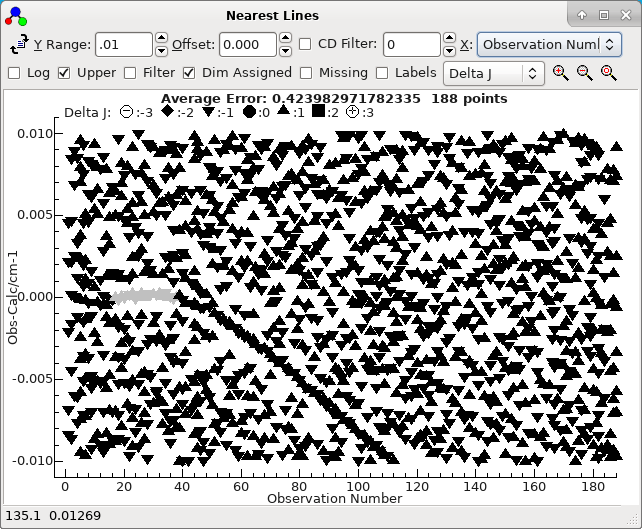The status bar displays the x, y co-ordinates of the mouse and, if the mouse is over an experimental point, the source of the data point. Right click on the point for various options for working on observations; see below for a list. If you select an area with a mouse additional options applying to the points inside or outside the area are available .If PGOPHER is correctly configured, you can go directly to the source of the data by right clicking on a given point and selecting "Edit Point" - see below for other options.

For the formulae below, oi is the ith observation, ci the corresponding calculated value, pi is a population and wi the weight. The population, pi, is the calculated population of the upper or lower state, depending on the setting of the Upper check box. The average error displayed at the top is simply [Σ[(oi-ci)/wi]2/nobs]½, which is slightly different to the value given in fits as it does not take account of the number of parameters floated. (This allows a value to be calculated without doing a fit.)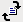If fitting using the Log Window, reload observations and recalculate plot.If fitting using the Line List Window, use the Test button instead.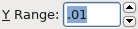Maximum distance to observed lines - controls vertical range of plot.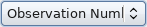Select the X axis of the plot. Possible choices are: Observation Number - i Frequency - The position of the transition; typically the same as oi, except for intensity fits. State Energy - The energy of the upper or lower state of the transition, depending on the Upper check box. Calculated - ci Observed - oi Weight - wi Population - pi Iobs - The observed intensity, if present in the fit input. Icalc - The calculated line intensity. J N F A: Ω for linear molecules, |K| for symmetric tops, Ka for asymmetric tops A + J/Jmax J + A/Amax B: F1, F2...for linear molecules, Kl for symmetric tops, Kc for asymmetric tops B + J/Jmax J + B/Bmax F1 Hyperfine quantum numbers (if appropriate) F2 ... F9 Note A and B above will normally show as the appropriate quantum number, unless multiple types of molecules are being simulated.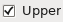Check to use upper (rather than lower) state quantum numbersfor the X axis.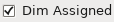Plot assignments grayed out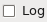Plot log10(Abs(X))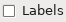Show filename changes when x axis is observation number and the observations are in multiple files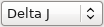Choose how to select the mark styles: Symmetry - A different mark for each state symmetry. Delta J - A different mark for each change in J. This is always upper J - lower J. Error Bars - plot wi as error bars.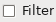Select plotted transitions using the Transition Selection and Details Window.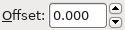Offset of plot window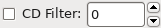Enable common difference filter - only plot transitions satisfying common difference to the tolerance set in the text box.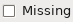Highlight lines with no possible assignment in range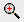Zoom plot to region selected with mouse.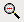Shrink plot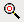Reset plot zoom to fill window